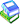This simple knowledge base derives the body-mass-index (BMI) of a person based on the weight and height. Please note that this is a simple example for demonstration uses!
To test it, add some values in the interview questionnaire below.

### Interview#

QuickInterview
Start questionaire
 Wie groß sind Sie? m
 Wie schwer sind Sie? kg
 Ihr Body Mass Index

ShowSolutions
-- no entries --

### The Knowledge Base#

In this part of the article, the knowledge base for this demo is defined.

#### Terminology#

We first define what questions can be asked and solutions can be derived in this demo.

Question
Start questionaire #1 - Height [num] (0.1 3) {m} - Weight [num] (1 300) {kg} - bmi [num] <abstract>

Solution
Weight Assessment
-
Underweight
-
Normal weight
-
Overweight
-
Severe overweight

We also define german question and solution texts. This is optional. If no texts are given for a certain language, the original name is shown.

Property
Height
.prompt.de = Wie groß sind Sie?
Weight
.prompt.de = Wie schwer sind Sie?
bmi
.prompt.de = Ihr Body Mass Index
Underweight
.prompt.de = Untergewicht
Normal weight
.prompt.de = Normalgewicht
Overweight
.prompt.de = Übergewicht
Severe overweight
.prompt.de = Starkes Übergewicht
Severe overweight
.description.de = Ihr BMI ist größer 35. Sie sollten dringend abnehmen.

#### Knowledge#

Here we define how the BMI is calculated and the solutions are derived.

The BMI is calculated using DiaFlux models, where first the "Height" and "Weight" is asked to the user. Then, the value of the abstraction "bmi" is derived, when values for the aforementioned questions are known. Final solutions are derived depending on the result of the calculated value of "bmi".

#### Definition of Knowledge Base#

Here we follow with some basic stuff that is needed to tell the system, that we have a knowledge base here. Using the menu that expands when hovering over the small book icon on the right, you can also export the knowledge base as a .d3web-file to load and run it in your own application (click Download), or start a one-choice-dialog for this demo (click Run Interview).KnowledgeBase
BMI Demo
BMIDemo

Package
BMIDemo